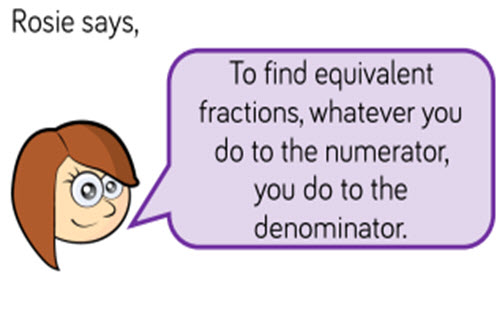# Fractions: applying the four operations

This guide provides a sequence of small steps for deepening understanding of fractions, from simplifying fractions to comparing and ordering fractions through to adding, subtracting, multiplying and dividing fractions.

Year level(s) Year 4, Year 5, Year 6
Audience Teacher
Purpose Teaching resource, Teaching strategies
Teaching strategies and pedagogical approaches Questioning, Explicit teaching
Keywords equivalent, simplify, improper fractions, mixed numbers, explicit teaching

## Curriculum alignment

Curriculum connections Numeracy
Strand and focus Number, Build understanding
Topics Addition and subtraction, Fractions, Multiplication and division, Operating with number
AC: Mathematics content descriptions
ACMNA077 Investigate equivalent fractions used in contexts
ACMNA078 Count by quarters halves and thirds, including with mixed numerals. Locate and represent these fractions on a number line
ACMNA102 Compare and order common unit fractions and locate and represent them on a number line
ACMNA103 Investigate strategies to solve problems involving addition and subtraction of fractions with the same denominator
ACMNA125 Compare fractions with related denominators and locate and represent them on a number line
ACMNA126 Solve problems involving addition and subtraction of fractions with the same or related denominators
ACMNA127 Find a simple fraction of a quantity where the result is a whole number, with and without digital technologies
National numeracy learning progression Additive strategies
Multiplicative strategies
Interpreting fractions - InF5, InF6, InF7